Technical Article

# Concepts and Variables with State-Space and Canonical Models

June 09, 2015 by Editorial Team

## An analysis of the concepts and variables associated with state-space, canonical and system transfer function representations of the common converters. In direct circuit linearization averaging technique, dynamic behavior of the circuit cannot be studied at the resonant frequency as the switching frequency component of the output for the resonant converter is different from the local average value frequency. Thus, the integral expression of the average value needs to be extended using the Fourier series; and the derivative expression with respect to a particular frequency is required for the dynamic analysis.

The Concepts and Variables Associated with State-Space, Canonical and System Transfer Function Representations of the Common Converters

Beginner

#### State-Space Averaging

In direct circuit linearization averaging technique, dynamic behavior of the circuit cannot be studied at the resonant frequency as the switching frequency component of the output for the resonant converter is different from the local average value frequency. Thus, the integral expression of the  average value needs to be extended using the Fourier series; and the derivative expression with respect to a particular frequency is required for the dynamic analysis.

Also, state-space model technique can be applied to continuous, discrete and sample data systems.

State-space models give a basic and powerful method for the dynamic modelling of numerous systems, such as the power converter. You can get the steady-state and the dynamic performances of the power processor, that has an open system or closed-loop system, by subjecting it to external disturbances. It also forms the base for the applications of nonlinear control methodology like the sliding-mode control.

The state-space averaging technique gives a complete converter model with steady-state and dynamic quantities.

Moreover, state- space modelling may be easier than the averaging by the direct formation of circuit studied earlier as the state-space models give a chance to organize the required computations. That is why there is a growing need of the state-space modelling in power electronics. Tools of classical LTI system control such as Bode Plot, root locus and etc is best suited with state-space average models.

#### State-Space Modelling

The main variables in a state-space model are called the state variables which define the state of the system. These variables abridge those aspects of the past that are related to the future. Thus, they are closely related to the memory or energy-storage mechanism. Other variables of interest are the signal input such as the source voltage and source current; and the control input such as the duty cycle ratio. These variables are also embodied in state-space model.

If the converter is operating in steady state without any external disturbances and if the switches are ideal i.e. no voltage drop during on-condition and no current during off-condition, then the behavior of the converter can be represented by the form of state-space model as,

$$\dot{x}=Ax+Bu$$

and

$$y=Cx+Du$$

Here, x is a state vector and u is the control or input vector,

$$\dot{x}=\frac{dx}{dt},$$

A, B, C, D are the state (or dynamics) matrix, input matrix, output matrix, and feed-forward (or direct transmission) matrix respectively.

The general solution for these state-space model equations is,

$$x(t)=e^{A(t-δT)}x(δT)+\int_{δT}^{α}e^{A(t-α)}B\;u(α)dα$$

If the limits of the time interval are between 0 to T, then the solution for the state-space model equation becomes,

$$x(t)=e^{A(t)}x(0)+\int_{0}^{T}e^{A(t-α)}B\;u(α)dα$$

If the switch in the converter is either in turn-on or turn-off condition represented by the time-variant switching variable δ(t), the state-space equation can be represented as,

For turn-on interval i.e. 0 ≤ t ≤ δ1t,

$$\dot{x}=A_{01}x+B_{01}u$$     [Equation 1]

$$y=C_{01}x+D_{01}u$$   [Equation 2]

Here, time-variant switching variable, δ(t) =1 for turn-on interval and for turn-off interval i.e. δ1t ≤ t ≤ T,

$$\dot{x}=A_{02}x+B_{02}u$$     [Equation 3]

$$y = C_{02}x+D_{02}u$$    [Equation 4]

Here, time-variant switching variable, δ(t) = 0 for turn off interval.

These equations can be combined to get the non-linear and time-variant state-space model as given below.

$$\dot{x}=[A_{01}δ(t)+A_{02}(1-δ(t))]x+[B_{01}δ(t)+B_{02}(1-δ(t))]u$$  [Equation 5]

$$y=[C_{01}δ(t)+C_{02}(1-δ(t))]x+[D_{01}δ(t)+D_{02}(1-δ(t))]u$$  [Equation 6]

The solution of these equations for time interval 0 to T is,

$$x(T)=e^{[A_{01}δ(t)+A_{02}(1-δ(t))]T}x(0)+\int_{0}^{T}e^{[A_{01}δ(t)+A_{02}(1-δ(t))](t-α)} [B_{01}δ(t)+B_{02}(1-δ(t))] u(α)dα$$

If δ1 is the average value and the average value of x is $$\overline{x}$$, then,

$$\dot{\overline{x}}=[A_{01}δ_{1}+A_{02}δ_{2}]\overline{x}+[B_{01}δ_{1}+B_{02}δ_{2}]\overline{u}$$   [Equation 7]

$$\overline{y}=[C_{01}δ_{1}+C_{02}δ_{2}]\overline{x}+[D_{01}δ_{1}+D_{02}δ_{2}]\overline{u}$$    [Equation 8]

Where, δ= 1-δ1

Method:

Replace the inductor with an equivalent current source and the capacitor by an equivalent voltage source as shown in Fig. 1. There must be some initial conditions for us know the output of the dynamic analysis. Note that state variables are those variables whose values are required to know the future system performance or behavior. Thus, inductor current and capacitor voltage are the usual choices for the state variable.

Find the inductor voltages and capacitor currents as the function of the source voltage or current, inductor current, capacitor voltage, and control inputs.

Also solve the output variables using step 2.

You can consider the switching function for the step 2 as given below.

$$v_{L}=\acute{f_{1}}(v_{s},i_{s},i_{L}, v_{c},q,t)$$

$$i_{C}=\acute{f_{2}}(v_{s},i_{s},i_{L}, v_{c},q,t)$$

$$y_{1}=n_{1}(v_{s},i_{s},i_{L}, v_{c},q,t)$$

$$y_{2}=n_{2}(v_{s},i_{s},i_{L}, v_{c},q,t)$$

Put the inductor voltage and capacitor current in the equations in step 2 and rearrange them to get the expressions for the derivative of the state variables. Finally, you can find the matrixes A, B , C and D.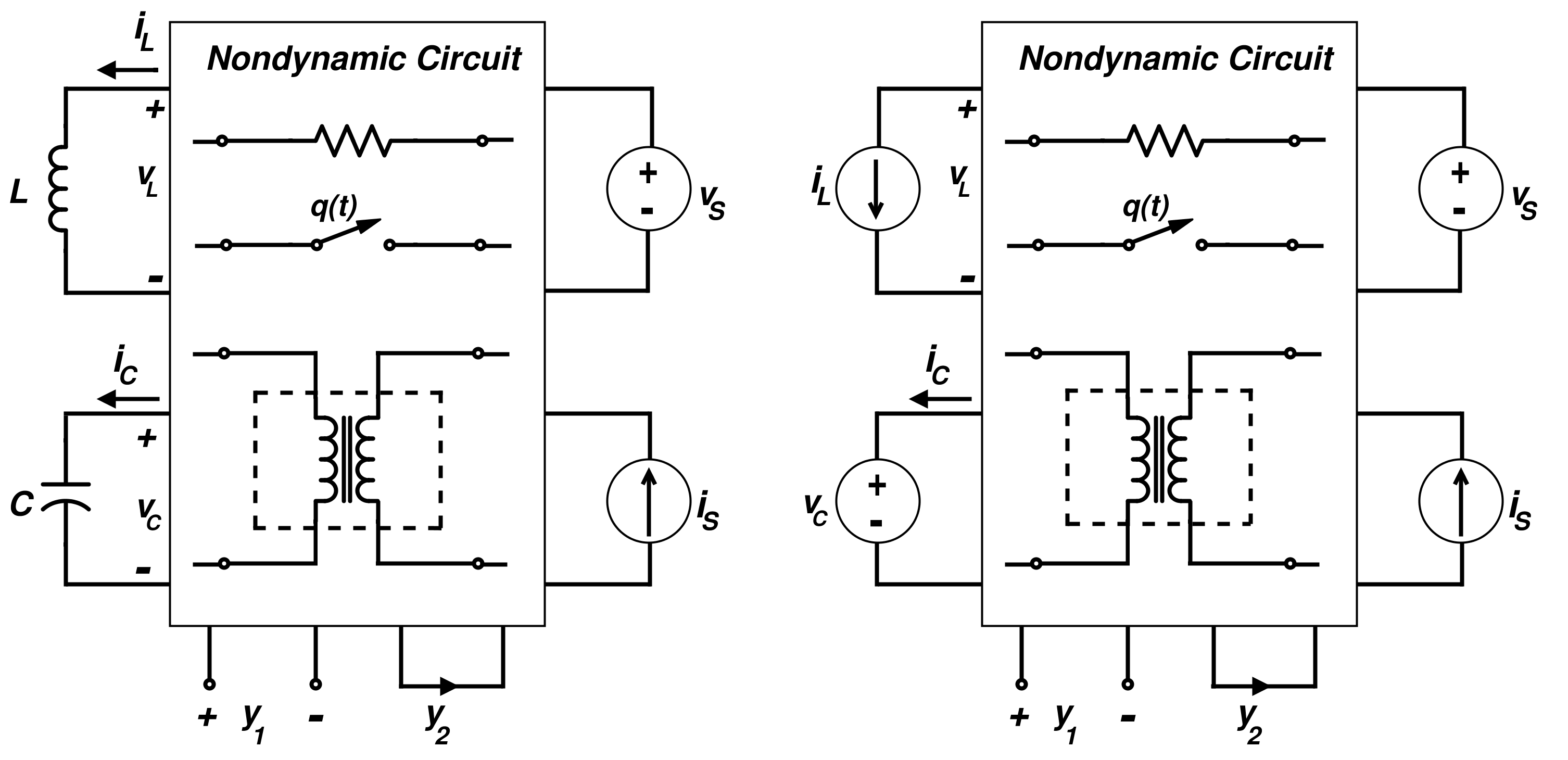Figure 1.  General Representation of the Converter Circuit

Here, the non-dynamic circuit consists of components such as resistor, switch, and an ideal transformer that have only instantaneous values of the voltages and currents i.e. they don’t have integral or derivative or time-shift components.

#### Linear State-Space Average Modelling

Consider there is a small disturbance in the system. Now, represent that small perturbation by a “ ̌ ” symbol above the letter and the steady state by a capital letter as done earlier.

Thus, we have,

$$\overline{x}=X+\check{x}$$

$$\overline{y}=Y+\check{y}$$

$$\overline{u}=U+\check{u}$$

$$δ_{1}=∆_{1}+\check{δ}$$

$$δ_{2}=∆_{2}-\check{δ}$$

$$\dot{X}=0$$

$$\Rightarrow \overline{\dot{x}}=\dot{X}+\check{x}$$

$$\Rightarrow \overline{\dot{x}}=\check{x}$$

Using Equations 7 and 8, we get,

$$\Rightarrow \overline{\dot{x}}=\check{x}=[A_{01}∆_{1}+A_{02}∆_{2}] X\,+\,[B_{01}∆_{1}+B_{02}∆_{2}] U\,+\,[A_{01}∆_{1}+A_{02}∆_{2}] \check{x}\,+\,[B_{01}∆_{1}+B_{02}∆_{2}] \check{u}+[(A_{01}-A_{02})X+(B_{01}-B_{02})U]\check{δ}\,+\,[(A_{01}-A_{02})\check{x}+(B_{01}-B_{02})\check{u}]\check{δ}$$

and
$$\overline{y}=Y+\check{y}=[C_{01}∆_{1}+C_{02}∆_{2}] X\,+\,[D_{01}∆_{1}+D_{02}∆_{2}] U\,+\,[C_{01}∆_{1}+C_{02}∆_{2}] \check{x}\,+\,[D_{01}∆_{1}+D_{02}∆_{2}] \check{u}\,+\,[(C_{01}-C_{02})X+(D_{01}-D_{02})U]\check{δ}\,+\,[(C_{01}-C_{02})\check{x}\,+\,(D_{01}-D_{02})\check{u}]\check{δ}$$

These equations can be separated into two parts, one is related to DC or steady-state behavior and other one is related to the linear small-signal state-space average model as given below:

$$[A_{01}∆_{1}+A_{02}∆_{2}] X\,+\,[B_{01}∆_{1}+B_{02}∆_{2}] U=0$$   [Equation 9]

and

$$Y=[C_{01}∆_{1}+C_{02}∆_{2}] X+[D_{01}∆_{1}+D_{02}∆_{2}] U$$ [Equation 10]

The steady-state representation of the average system although linear, they are not time-invariant as these are dependent on ∆ . Linear system helps us to define the different transfer functions which can be used to design the closed-loop system controller further.

#### $$\overline{\dot{x}}=\check{x}=[A_{01}∆_{1}+A_{02}∆_{2}] \check{x}\,+\,[B_{01}∆_{1}+B_{02}∆_{2}] \check{u}\,+\,[(A_{01}-A_{02})X+(B_{01}-B_{02})U]\check{δ}\,+\,[(A_{01}-A_{02})\check{x}+(B_{01}-B_{02})\check{u}]\check{δ}$$

If we neglect the higher-order term

$$[(A_{01}-A_{02})\check{x}+(B_{01}-B_{02})\check{u}]\check{δ}=0,$$

then,

$$\overline{\dot{x}}=\check{x}=[A_{01}∆_{1}+A_{02}∆_{2}] \check{x}\,+\,[B_{01}∆_{1}+B_{02}∆_{2}] \check{u}\,+\,[(A_{01}-A_{02})X+(B_{01}-B_{02})U]\check{δ}$$

and

$$\check{y}=[C_{01}∆_{1}+C_{02}∆_{2}] \check{x}\,+\,[D_{01}∆_{1}+D_{02}∆_{2}] \check{u}\,+\,[(C_{01}-C_{02})X+(D_{01}-D_{02})U]\check{δ}\,+\, [(C_{01}-C_{02})\check{x}+(D_{01}-D_{02})\check{u}]\check{δ}$$

Neglecting the higher-order term, we get,

$$\check{y}=[C_{01}∆_{1}+C_{02}∆_{2}] \check{x}\,+\,[D_{01}∆_{1}+D_{02}∆_{2}] \check{u}\,+\,[(C_{01}-C_{02})X+(D_{01}-D_{02})U]\check{δ}\,+\, [(C_{01}-C_{02})\check{x}$$

This can also be written as given below.

$$\dot{\check{x}}=A_{Avg} \check{x}\,+\,B_{Avg} \check{u}\,+\,[(A_{01}-A_{02})X+(B_{01}-B_{02})U]\check{δ}$$   [Equation 11]

and

$$\check{y}=C_{Avg} \check{x}\,+\,D_{Avg} \check{u}\,+\,[(C_{01}-C_{02})X\,+\,(D_{01}-D_{02})U]\check{δ}$$   [Equation 12]

Where:

$$A_{Avg}=A_{01}∆_{1}+A_{02}∆_{2}$$

$$B_{Avg}=B_{01}∆_{1}+B_{02}∆_{2}$$

$$C_{Avg}=C_{01}∆_{1}+C_{02}∆_{2}$$

$$D_{Avg}=D_{01}∆_{1}+D_{02}∆_{2}$$     [Equation 13]

#### Transfer Function for Converter

Transfer function for the converter can be readily obtained with the help of the Laplace transform. These are helpful for the dynamic analysis of the converter. The transient response, due to input disturbances, can be found out using the input-susceptibility function.

Under steady-state condition, Equations 9 and 10, we get,

$$\frac{Y}{U}=-C_{Avg}A^{-1}_{Avg}B_{Avg}+D_{Avg}$$      [Equation 14]

Assume the zero initial condition $$(\check{u}=0)$$, by using Laplace transform for the Equations 11 and 12, we get,

Control Transfer Function

$$\frac{\check{y}(s)}{\check{δ}(s)}={C_{Avg}[sI-A_{Avg}]}^{-1}[(A_{01}-A_{02})X+(B_{01}-B_{02})U]+[(C_{01}-C_{02})X+(D_{01}-D_{02})U]$$[Equation 15]

Considering that there is a small signal disturbance such that it can be assumed that $$\check{δ}=0,$$

Then, the transfer function (or audio susceptibility) is given by

$$\frac{\check{y}(s)}{\check{δ}(s)}={C_{Avg}[sI-A_{Avg}]}^{-1}B_{Avg}+D_{Avg}$$   [Equation 16]

Now, consider the example of the buck-boost converter and the forward converter for the state-space average modelling.

State-Space Averaging for the Buck-Boost Converter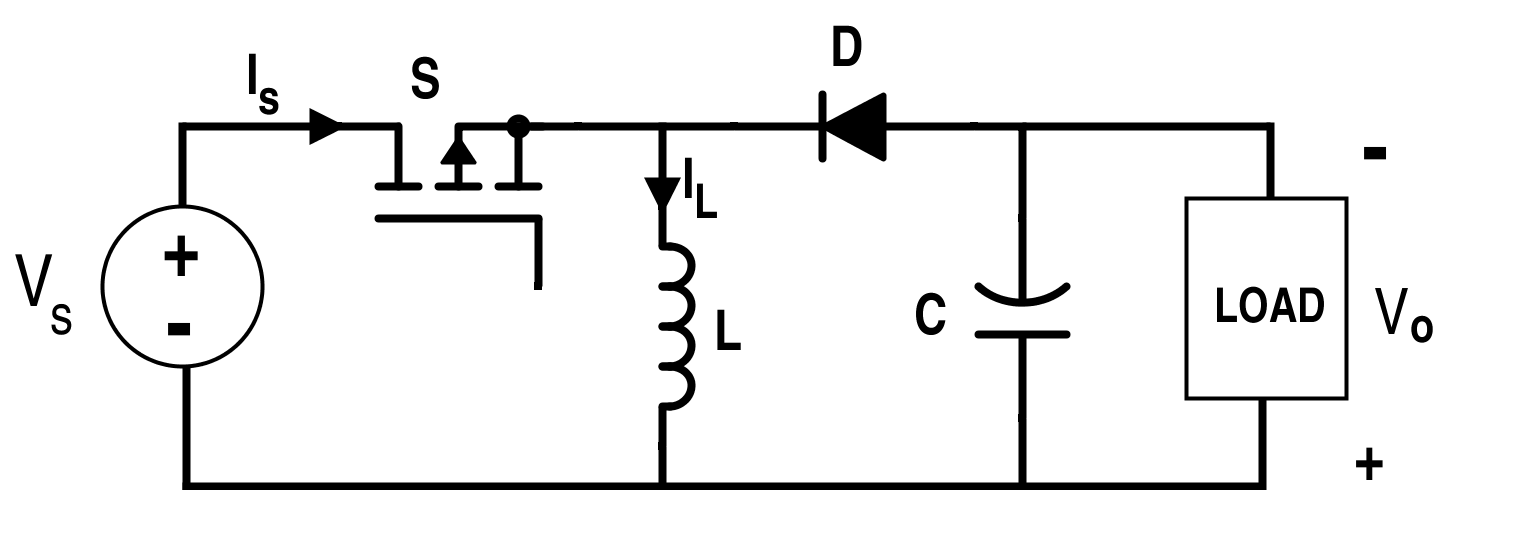Figure 2. Circuit for the Non-Isolated Buck-Boost Converter

State Vector,

$$x=[I_{L}\;V_{C}]$$

The equations when the switch S is in ON-condition:

$$V_{s}=L\frac{di_{L}}{dt}$$

$$\Rightarrow \frac{di_{L}}{dt}=\frac{V_{S}}{L}$$

also,

$$C\frac{dv_{o}}{dt}=-\frac{v_{o}}{R}$$

and

$$v_{o}=-v_{c}$$

The equations when the switch S is in OFF-condition:

$$\frac{di_{L}}{dt}=-\frac{v_{o}}{L}$$

$$\frac{dv_{c}}{dt}=\frac{i_{L}}{C}-\frac{v_{o}}{RC}$$

and

$$v_{o}=-v_{C}$$

consider

$$y = [v_{o}\;i_{L}]$$

Comparing it with the general state-space equations from (1) to (4), we get,

$$A_{01}=[0\;0\;0\;-\frac{1}{RC}]$$

$$A_{02}=[0\;-\frac{1}{L}\;\frac{1}{C}\;-\frac{1}{RC}]$$

$$B_{01}=[\frac{1}{L}\;0]$$

$$B_{02}=[0\;0]$$

$$C_{01}=[0\;1\;1\;0]$$

$$C_{02}=[0\;1\;1\;0]$$

$$D_{01}=[0\;0]$$

$$D_{02}=[0\;0]$$

Using Equations 5 and 6, we can get the switched state model as given below.

$$[\dot{i_{L}}\;\dot{v_{o}}]=[0\;-\frac{1-δ(t)}{L}\;\frac{1-δ(t)}{C}-\frac{1}{RC}][i_{L}\;v_{o}]+[\frac{δ(t)}{L}\;0]v_{s}$$

and

$$[v_{o}\;i_{L}]=[0\;1\;1\;0][i_{L}\;v_{o}]+[0\;0]v_{s}$$

Now, if we apply the Equations 7 and 8, we can get the following equations for the  average state-space:

$$[\overline{\dot{i_{L}}}\;\overline{\dot{v_{o}}}]=[[0\;0\;0\;-\frac{1}{RC}]δ_{1}+[0-\frac{1}{L}\frac{1}{C}-\frac{1}{RC}]δ_{2} [\overline{i_{L}}\;\overline{v_{o}}]+[[\frac{1}{L}\;0]δ_{1}+[0\;0]δ_{2}]\overline{v_{s}}$$

and

$$[\overline{v_{o}}\;\overline{i_{L}}]=[[0\;1\;1\;0]δ_{1}+[0\;1\;1\;0]δ_{2}]\,[\overline{i_{L}}\;\overline{v_{o}}]+[[\frac{1}{L}\;0]δ_{1}+[0\;0]δ_{2}]\overline{v_{s}}$$

As we know, δ= 1-δ1

We get,

$$[\dot{\overline{i_{L}}}\;\dot{\overline{v_{o}}}]=[0\;-\frac{1-δ_{1}(t)}{L}\;\frac{1-δ_{1}(t)}{C}\;-\frac{1}{RC}]\;[\overline{i_{L}}\;\overline{v_{o}}]+[\frac{δ_{1}(t)}{L}\;0]\overline{v_{s}}$$    [Equation 17]

and

$$[\overline{v_{o}}\;\overline{i_{L}}]=[0\;1\;1\;0]\;[\overline{i_{L}}\;\overline{v_{o}}]+[0\;0]\overline{v_{s}}$$     [Equation 18]

The characteristics roots of the matrix A are the roots of |sI - A|. Thus,

$$s_{1,2}=\sqrt{-\frac{1}{2RC}\;±\;\frac{{(1-δ_{1})}^{2}}{LC}}$$

The maximum value of the root s is valid for switching frequency fs,  sT << 1, for the linear approximation.  smax can be regarded as a frequency which is much less than 2 π fs.

For instance, it can be considered at one-tenth of the switching frequency. Then, we have to choose the inductor and capacitor values accordingly.

Using Equations 11, 12 and 13, we have,

Linear state-space model for this converter is given by

$$[\check{i_{L}}\;\check{v_{o}}]=[0\;-\frac{1-∆_{1}}{L}\frac{1-∆_{1}}{C}-\frac{1}{RC}][\check{i_{L}}\;\check{v_{o}}]\;+\;[\frac{∆_{1}}{L}\;0][\check{v_{s}}]\;+\;[0\;\frac{\check{δ}}{L}-\frac{\check{δ}}{C}\;0][\check{i_{L}}\;\check{v_{o}}]\;+\;[\frac{V_{S}}{L}\;0][\check{δ}]$$

and

$$[\check{v_{o}}\;\check{i_{L}}]=[0\;1\;1\;0][\check{i_{L}}\;\check{v_{o}}]+[0\;0][\check{v_{s}}]$$

Thus, we have the following matrixes:

$$A_{Avg}=[0\;-\frac{1-∆_{1}}{L}\frac{1-∆_{1}}{C} -\frac{1}{RC}]$$

$$B_{Avg}=[\frac{∆_{1}}{L}\;0]$$

$$C_{Avg}=[0\;1\;1\;0]$$

$$D_{Avg}=[0\;0]$$

Using Equation 14 and these above matrixes, we get,

$$\frac{I}{V_{S}}=\frac{∆_{1}}{{R(∆_{1}-1)}^{2}}$$

and

$$\frac{V_{O}}{V_{S}}=\frac{∆_{1}}{∆_{1}-1}$$ (for steady-state case of buck-boost converter)

Now, for zero small-signal duty cycle disturbances, transfer function using Equation 16, we can get the following:

$$\frac{\check{y}(s)}{\check{δ}(s)}=C_{Avg}{[sI-A_{Avg}]}^{-1}B_{Avg}+D_{Avg}$$

$$\Rightarrow \frac{\check{i_{L}}(s)}{\check{V_{S}}(s)}=\frac{∆_{1}(1+sCR)}{{s}^{2}LCR+sL+{R(1-∆1)}^{2}}$$

and

$$\frac{\check{v_{o}}(s)}{\check{v_{o}}(s)}=R\frac{∆_{1}(1-∆_{1})}{{s}^{2}LCR+sL+{R(1-∆1)}^{2}}$$

The control transfer functions i.e. ratio of the output to small-signal duty cycle by using Equation 15 are:

$$\frac{\check{i_{L}}(s)}{\check{δ}(s)}=\frac{V_{S}(\frac{1+∆_{1}+sCR}{1-∆_{1}})}{{s}^{2}LCR+sL+{R(1-∆1)}^{2}}$$

and

$$\frac{\check{v_{o}}(s)}{\check{δ}(s)}=\frac{V_{S}(R-\frac{sL∆_{1}}{{(1-∆_{1})}^{2}})}{{s}^{2}LCR+sL+{R(1-∆_{1})}^{2}}$$

#### The Canonical Circuit Model for Buck-Boost Converter

From the Buck-Boost Converter state-space average model given in Equations 17 and 18, we can get the following canonical model of it as shown in Fig. 3.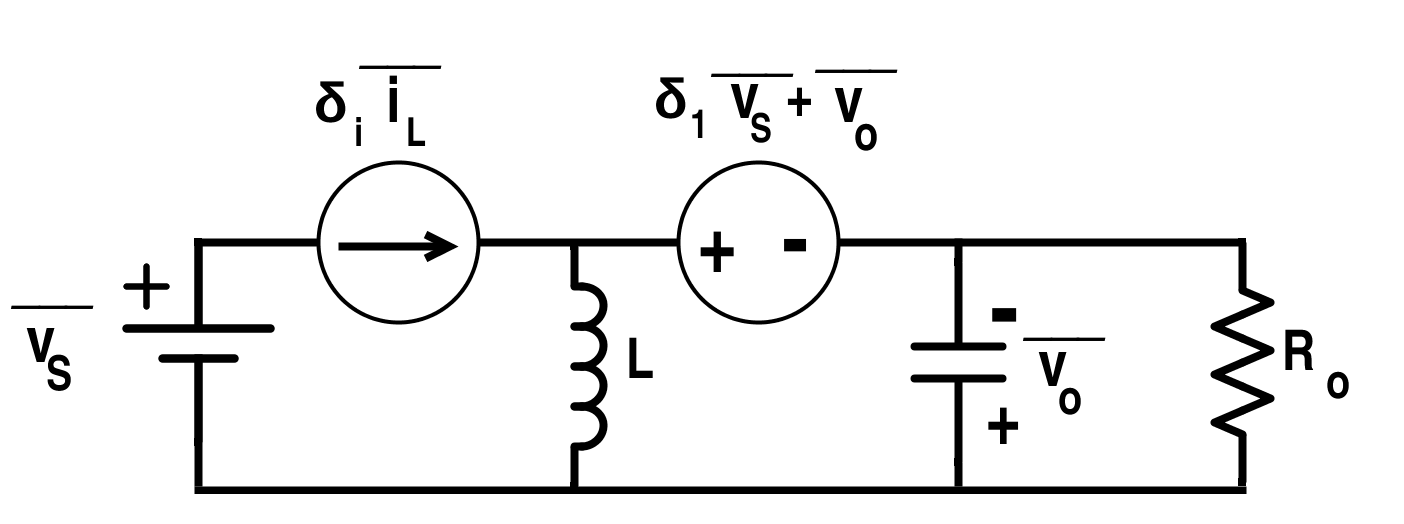Figure 3. Canonical Circuit of the Buck-Boost Converter for the Average State-Space Model

#### State-Space Averaging for the Non-Ideal Forward Converter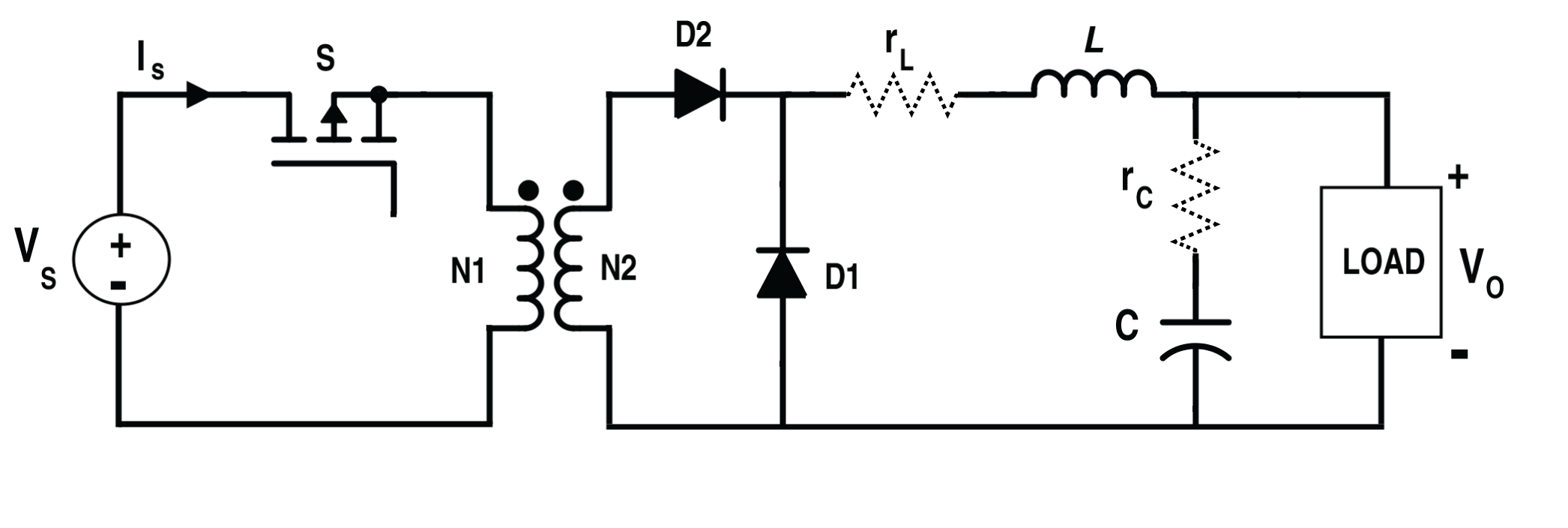Figure 4. Circuit Diagram of the Non-Ideal Forward Converter

State Vector,

$$x=[i_{L}\;v_{c}]$$

Output Vector,

$$y=[i_{L}\;v_{o}]$$

$$U=[V_{S}]$$

Turns Ratio,

$$n=\frac{N2}{N1}$$

For the time interval 0 ≤ t ≤ δ1T,

Time-variant switching variable, δ (t) =1 for turn-on interval.

During this interval switch S and D1 are in on-condition while D2 is in off-condition.

And for the time interval δ1T ≤ t ≤ T,

Time-variant switching variable, δ (t) =0 for turn-off interval.

During this interval switch S and D1 are in off-condition while D2 is in on-condition.

Switch State-Space model equations will be,

$$\frac{di_{L}}{dt}=-\frac{R\;rc+R\;rL+rL\;rC}{L(R+rc)}-\frac{R}{L(R+rc)v_{c}}+δ(t)nV_{S}$$

$$\frac{dv_{c}}{dt}=\frac{R}{(R+rc)C}i_{L}-\frac{1}{(R+r_{C})C}v_{c}$$

$$v_{o}=\frac{rc}{1+\frac{rc}{R}}i_{L}+\frac{1}{1+\frac{rc}{R}}v_{c}$$
Let

$$rm=\frac{rc}{1+\frac{rc}{R0}},$$ $$Rc=R+rc\,,\,Kc=\frac{R}{Rc}\,,\,rp=rL+rm$$

Then,

$$A_{01}=A_{02}=[-\frac{rp}{L}-\frac{Kc}{L}\;\frac{Kc}{C}-\frac{1}{RC}]$$

$$B_{01}=[\frac{n}{L}\;0]$$

$$B_{02}=[0\;0]$$

$$C_{01}=C_{02}=[1\;0\;rm\;Kc]$$

$$D_{01}=D_{02}=[0\;0]$$

Thus, the state-space average model is,

$$[\overline{\dot{i_{L}}}\;\overline{\dot{v_{C}}}]=[-\frac{rp}{L}-\frac{Kc}{L}\;\frac{Kc}{C}-\frac{1}{RC}][\overline{i_{L}}\;\overline{v_{c}}]+[\frac{nδ_{1}}{L}\;0][\overline{V_{S}}]$$
and

$$[\overline{i_{L}}\;\overline{v_{o}}]=[1\;0\;rm\;Kc]+[0\;0][V_{S}]$$

The canonical or equivalent circuit arising from these two matrixes is shown below.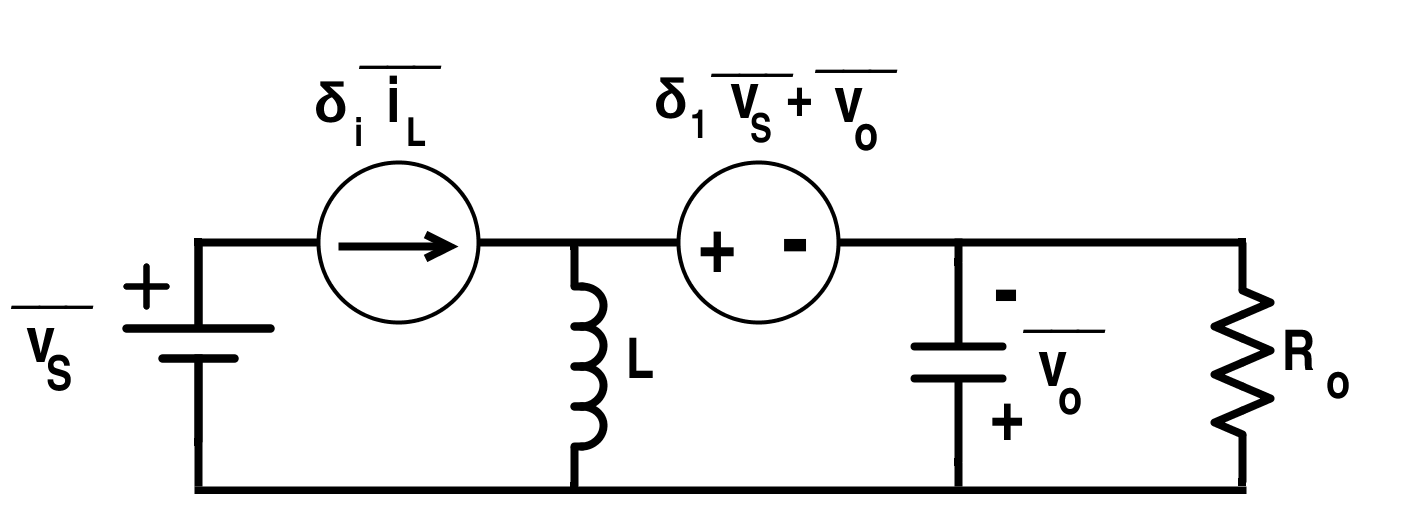Figure 5. Canonical Circuit of the Forward Converter for the Average State-Space Model

The Eigen value for this converter is,

$$s_{1,2}=-\frac{L+CRc\;rp±\sqrt{(-4Rc\;L\;C(Rc\;{Kc}^{2}+rp)+{(L+C Rc\;rp)}^{2})}}{2Rc\;L\;C}$$

Using Equations 11 and 12, we get,

$$[\dot{\check{i_{L}}}\;\dot{\check{v_{c}}}]=[-\frac{rp}{L}\;-\frac{Kc}{L}\;\frac{Kc}{C}\;-\frac{1}{RC}][\check{i_{L}}\;\check{v_{c}}]+[\frac{n∆_{1}}{L}\;0][\check{v_{s}}]+[\frac{nV_{S}}{L}\;0][\check{δ}]$$

and

$$[\check{i_{L}}\;\check{v_{o}}]=[1\;0\;rm\;Kc][\check{i_{L}}\;\check{v_{c}}]+[0\;0][V_{S}]$$

#### Transfer Function for Forward Converter

$$\frac{i_{L}}{V_{S}}=\frac{n∆_{1}}{{Kc}^{2}Rc+rp}$$

and

$$\frac{V_{O}}{V_{S}}=\frac{n∆_{1}({Kc}^{2}Rc+rm)}{{Kc}^{2}Rc+rp}$$

Small-signal input transfer functions

$$\frac{\check{i_{L}}(s)}{\check{v_{s}}(s)}=n\frac{∆_{1}(1+sCRc)}{{s}^{2}LC\;Rc+s(L+C\;Rc\;rp)+{Kc}^{2}Rc+rp}$$

and

$$\frac{v_{o}(s)}{v_{s}(s)}=n\frac{∆_{1}({Kc}^{2}Rc+rm+sC\;Rc\;rm)}{{s}^{2}LC\;Rc+s(L+C\;Rc\;rp)+{Kc}^{2}Rc+rp}$$

Small-signal control transfer functions,

$$\frac{\check{i_{L}}(s)}{\check{δ}(s)}=n\frac{V_{S}(1+s C\;Rc)}{{s}^{2}LC\;Rc+s(L+C\;Rc\;rp)+{Kc}^{2}Rc+rp}$$

and
$$\frac{v_{o}(s)}{δ(s)}=n\frac{V_{S}({Kc}^{2}Rc+rm+sCRc\;rm)}{{s}^{2}LC\;Rc+s(L+C\;Rc\;rp)+{Kc}^{2}Rc+rp}$$

Similar analysis can be done for other converters and some particular deviations can be considered for the formation of small-signal state-space model as shown below for buck converter. Also, these models can be formed for the discontinuous-conduction mode if required.

#### State-Space Averaging for the Buck Converter

The circuit for non-isolated ideal buck converter is shown in Fig.6.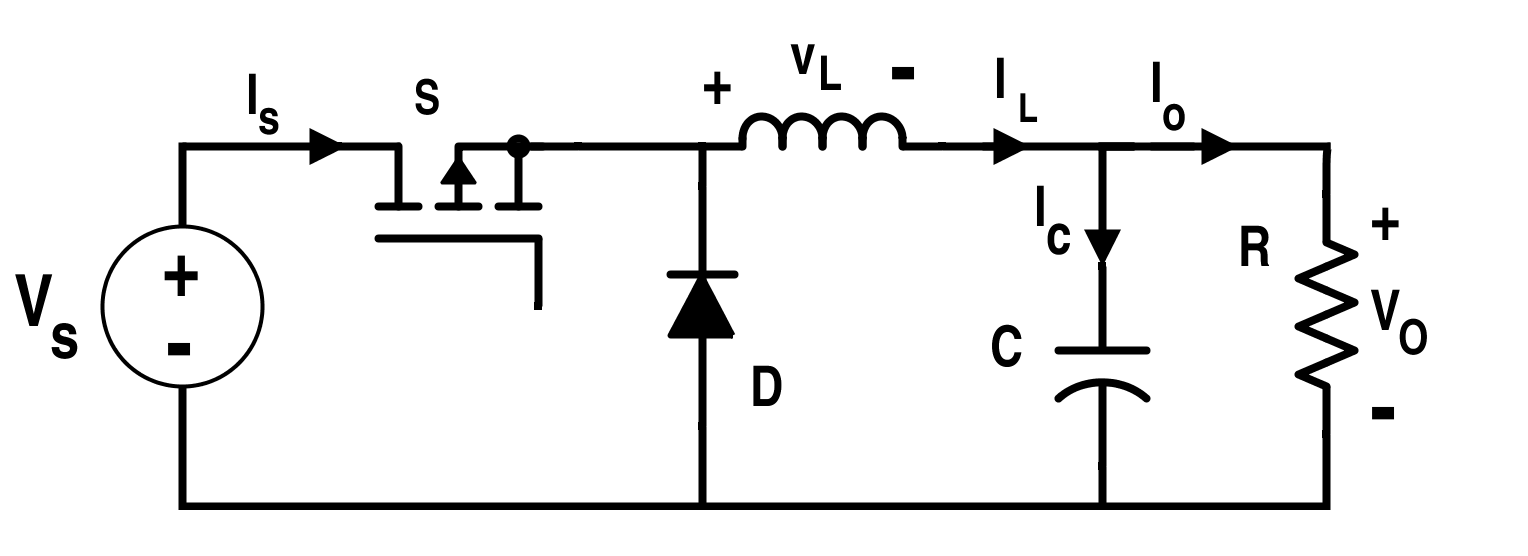Figure 6. Circuit of an Ideal Buck Converter

When the switch S in ON-condition, the equations are,

$$V_{S}=L\frac{di_{L}}{dt}+V_{C}$$

$$\Rightarrow \frac{di_{L}}{dt}=\frac{V_{S}-V_{C}}{L}$$

also,

$$i_{L}=C\frac{dv_{c}}{dt}+\frac{v_{c}}{R}$$

And vo=vc

The equations when the switch S is in OFF-condition:

$$\frac{di_{L}}{dt}=-\frac{v_{c}}{L}$$

$$\frac{dv_{c}}{dt}=\frac{i_{L}}{C}-\frac{v_{c}}{RC}$$

And vo=vc

$$\dot{x}=[\frac{di_{L}}{dt}\;\frac{dv_{c}}{dt}]$$

Comparing these equations with the state-space equations from (1) to (4), we get,

*Note: The D matrix is null as there is no feed-forward path.

$$A_{01}=A_{02}=[0\;-\frac{1}{L}\;\frac{1}{C}\;-\frac{1}{RC}]$$

$$B_{01}=[\frac{1}{L}\;{0}]$$

$$B_{02}=[0\;0]$$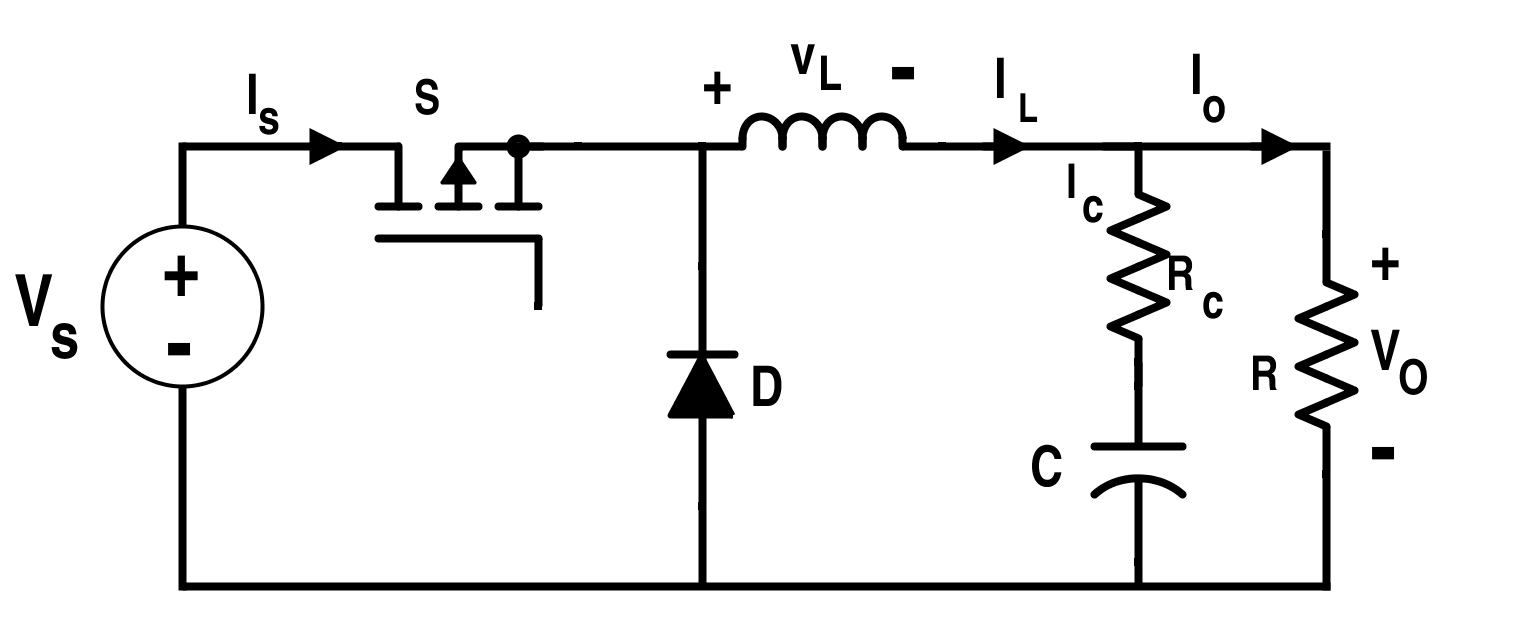Figure 7. Circuit of the Buck Converter with Non-Ideality

Considering the non-ideality such that a capacitor has some resistance which is represented by the equivalent resistance in series of the capacitor, RC. In that case, matrixes will be changed.

Then the small-signal transfer functions will be:

Small-signal control transfer function,

$$\frac{\check{v_{o}}(s)}{\check{δ}(s)}=\frac{RV_{S}(1+sRc\;C)}{R+s(L+R\;Rc\;C)+{s}^{2}(R+Rc)LC}$$

and

Small-signal input transfer function,

$$\frac{\check{v_{o}}(s)}{\check{v_{s}}(s)}=\frac{R∆_{1}(1+sRc\;C)}{R+s(L+R\;Rc\;C)+{s}^{2}(R+Rc)LC}$$

If the buck converter is acting in discontinuous-conduction mode, there will be three intervals in one switching period; and the average state-space coefficient matrix will be expressed as given below.

$$\overline{A}=A_{1}d_{1}+A_{2}d_{2}+A_{3}(1-d_{1}-d_{2})$$

$$\overline{B}=B_{1}d_{1}+B_{2}d{2}+B_{3}(1-d_{1}-d_{2})$$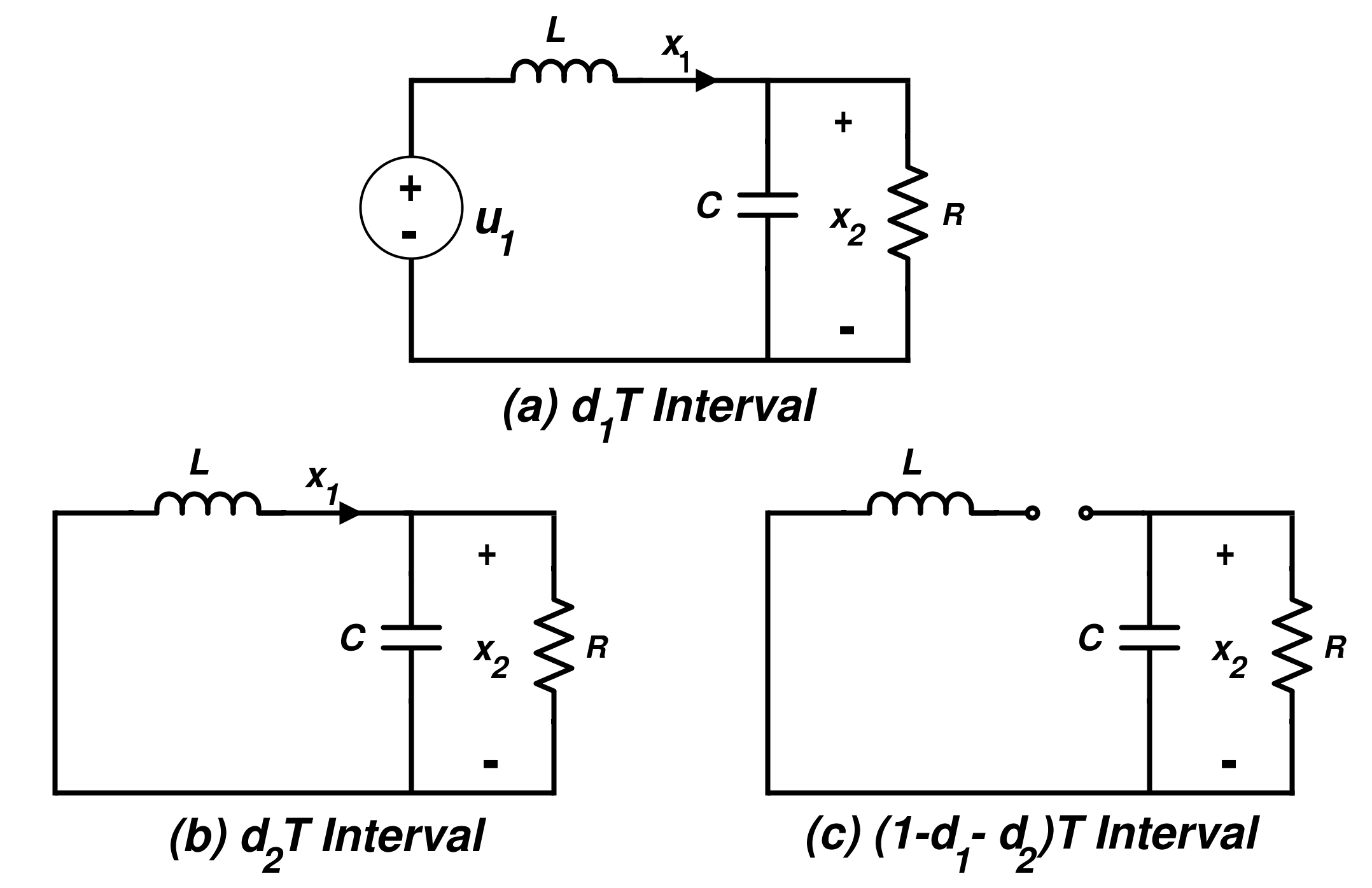Figure 8. Switching States of the Buck Converter in Discontinuous Conduction Mode

In discontinuous conduction mode for the buck converter, average state-space equation in the matrix form is shown below.

$$[\overline{\dot{x_{1}}}\;\overline{\dot{x_{2}}}]=[0\;-\frac{d_{1}+d_{2}}{L}\;\frac{d_{1}+d_{1}}{C}-\frac{1}{RC}][x_{1}\;x_{2}]+[\frac{d_{1}}{L}\;0]u_{1}$$

#### The Disadvantage of State-Space Averaging Technique

This state-space model may not be able to indicate the “sparsity” of the interconnection. However, it is not a concern for power electronic circuits as compared to large-power systems. In cases where it is considered a concern, generalized technique for the state-space model can be implemented.